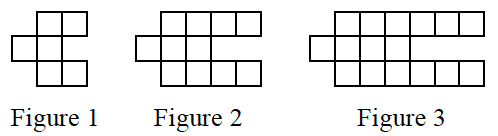### Home > CCA > Chapter 1 > Lesson 1.2.1 > Problem1-37

1-37.Examine the tile pattern shown at right. 1-37 HW eTool (CPM). Homework Help ✎

1. On graph paper, draw Figure 0 and Figure 4.

Use the eTool below to complete the tile pattern for Figure 0 and Figure 4.
Click the link at right for a full version of the eTool: CCA 1-37 HW eTool

2. How many tiles will Figure 10 have? How do you know?

• Find the equation for the pattern. Making a table can be helpful.
How many tiles does the pattern grow by in each figure?